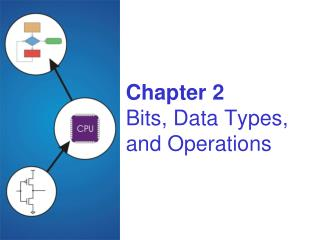DownloadDownload PresentationChapter 2 Bits, Data Types, and Operations

Chapter 2 Bits, Data Types, and Operations

Download PresentationChapter 2 Bits, Data Types, and Operations

- - - - - - - - - - - - - - - - - - - - - - - - - - - E N D - - - - - - - - - - - - - - - - - - - - - - - - - - -
Presentation Transcript

1. Chapter 2Bits, Data Types,and Operations

2. Hexadecimal Notation • It is often convenient to write binary (base-2) numbersas hexadecimal (base-16) numbers instead. • fewer digits -- four bits per hex digit • less error prone -- easy to corrupt long string of 1’s and 0’s

3. Convert Hexadecimal (2’s C binary) to Decimal • Given a hex digit that represents 2’s complement binary, convert into a decimal. • Example: 6Fhex or x6F • Determine the sign of the number. If the msh (most significant hex) value is 8 or greater then the sign is negative.6Fhex, sign + b/c msh (6) < 8sign positive • Use positional notation to convert6x161 + Fx160 = 6x161 + 15 = 96 + 15 = 1116Fhex = 111ten

4. Convert Hexadecimal (2’s C binary) to Decimal • Given a hex digit that represents 2’s complement binary, convert into a decimal. • Example: A0Fhex or xA0F • Determine the sign of the number. If the msh (most significant hex) value is 8 or greater then the sign is negative.A0Fhex, sign - b/c msh (A) < 8sign negative • Since negative, must apply 2’s complement • Convert to signed magnitude to decimal with positional notation-(5x162 + Fx161 + 1x160) = -(5x256 + 15x16 + 1) = -1,521 5F0+ 1 5F1 FFF- A0F 5F0 A0F= - 5F1